Open in App
Not now

# Class 8 RD Sharma Solutions – Chapter 25 Data Handling III (Pictorial Representation Of Data As Pie Charts Or Circle Graphs) – Exercise 25.1 | Set 1

• Last Updated : 13 May, 2021

### Present the information in the form of a pie-chart.

Solution:

Given that, total number of hours = 24

Therefore,

Central angle = (component value / 24) × 360°

Now the central angle for each activity will be calculated as follows :

Steps to construct pie chart using above data:

Step 1. Draw the circle of an appropriate radius.

Step 2. Draw a vertical radius anywhere in the circle.

Step 3. Now choose the largest central angle here, it is 120o then Construct a sector of central angle 120o whose one radius coincides with the radius drawn in step 2 and the other radius is in clockwise direction to the vertical radius.

Step 4. Construct other sectors representing other values in clockwise direction in descending order of values of their central angles.

Step 5. Shade the sectors so obtained by different colors and label them.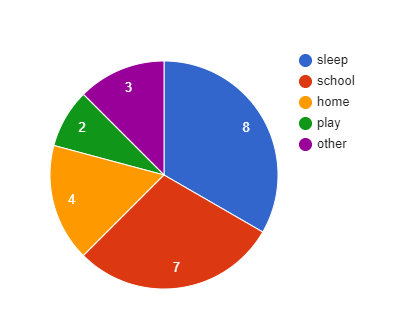Question 2. Employees of a company have been categorized according to their religions as given below :

### Draw a pie-chart to represent the above information.

Solution:

Given that, total number of workers = 1080

Therefore,

Central angle = (component value / 1080) × 360°

Now the central angle for each activity will be calculated as follows :

Steps to construct pie chart using above data :

Step 1. Draw the circle of an appropriate radius.

Step 2. Now draw a vertical radius anywhere inside the circle.

Step 3. Now choose the largest central angle. Construct a sector of central angle, whose one radius coincides with the radius drawn in step 2 and the other radius is in clockwise direction to the vertical radius.

Step 4. Construct other sectors representing other values in clockwise direction in descending order of values of their central angles.

Step 5. Shade the sectors so obtained by different colors and label them.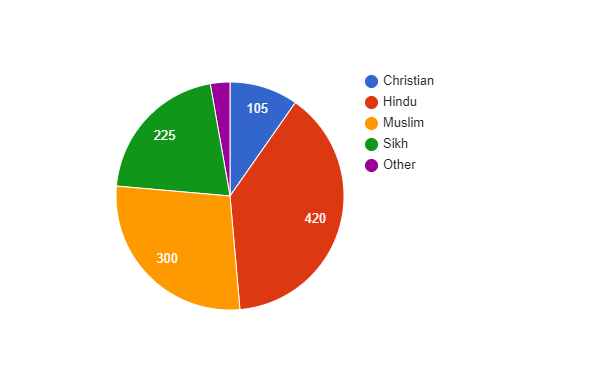### Draw a pie-chart to represent the above information.

Solution:

Given that, total sales = 480

Therefore,

Central angle = (component value/480) × 360°

Now the central angle for each activity will be calculated as follows :

Steps to construct pie chart using above data :

Step 1. Draw the circle of an appropriate radius.

Step 2. Now draw a vertical radius anywhere inside the circle.

Step 3. Now choose the largest central angle. Construct a sector of central angle, whose one radius coincides with the radius drawn in step 2 and the other radius is in clockwise direction to the vertical radius drawn in step 2.

Step 4. Construct other sectors representing other values in clockwise direction in descending order of values of their central angles.

Step 5. Shade the sectors so obtained by different colors and label them.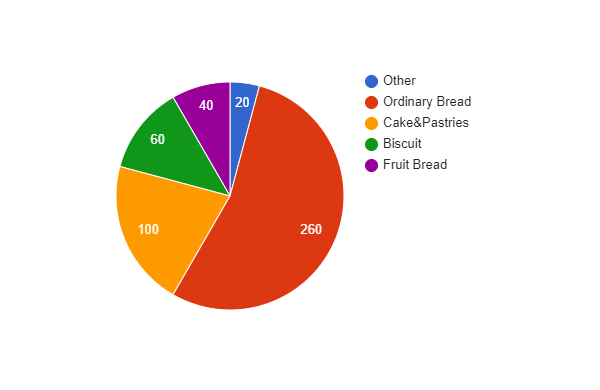### Question 4. The following data shows the expenditure of a person on different items during a month. Represent the data by a pie-chart.

Solution:

Given that, total amount = 10800 Rs

Therefore,

Central angle = (component value/10800) × 360°

Now the central angle for each activity will be calculated as follows :

Steps to construct pie chart using above data :

Step 1. Draw the circle of an appropriate radius.

Step 2. Now draw a vertical radius anywhere inside the circle.

Step 3. Choose the largest central angle. Construct a sector of central angle, whose one radius coincides with the radius drawn in step 2 and the other radius is in clockwise direction to the vertical radius.

Step 4. Construct other sectors representing other values in clockwise direction in descending order of values of their central angles.

Step 5. Shade the sectors so obtained by different colors and label them.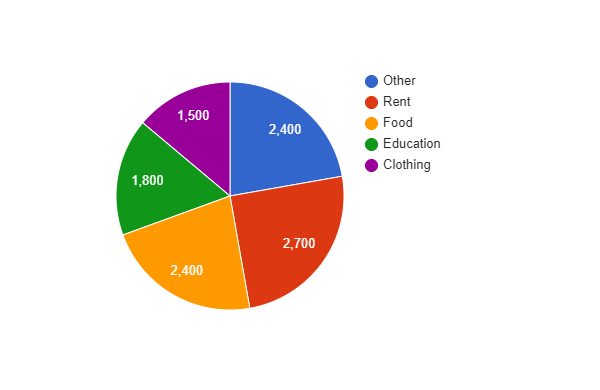### Present the information in the form of a pie-chart.

Solution:

Given that, total % of workers = 100%

Therefore,

Central angle = (component value/100) × 360°

Now the central angle for each activity will be calculated as follows :

Steps to construct pie chart using above data :

Step 1. Draw the circle of an appropriate radius.

Step 2. Now draw a vertical radius anywhere inside the circle.

Step 3. Now choose the largest central angle. Construct a sector of central angle, whose one radius coincides with the radius drawn in step 2 and the other radius is in clockwise direction to the vertical radius.

Step 4. Construct other sectors representing other values in clockwise direction in descending order of values of their central angles.

Step 5. Shade the sectors so obtained by different colors and label them.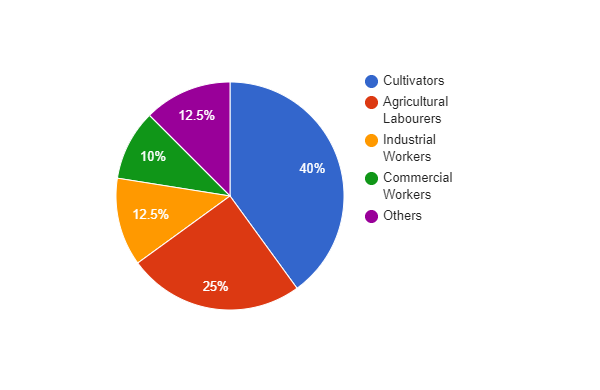### Present the above data in the form of pie-chart.

Solution:

Given that, total Expenditure (in %) = 100%

Therefore,

Central angle = (component value/100) × 360°

Now the central angle for each activity will be calculated as follows :

Steps to construct pie chart using above data :

Step 1. Draw the circle of an appropriate radius.

Step 2. Now draw a vertical radius anywhere inside the circle.

Step 3. Now choose the largest central angle. Construct a sector of central angle, whose one radius coincides with the radius drawn in step 2 and the other radius is in clockwise direction to the vertical radius.

Step 4. Construct other sectors representing other values in clockwise direction in descending order of values of their central angles.

Step 5. Shade the sectors so obtained by different colors and label them.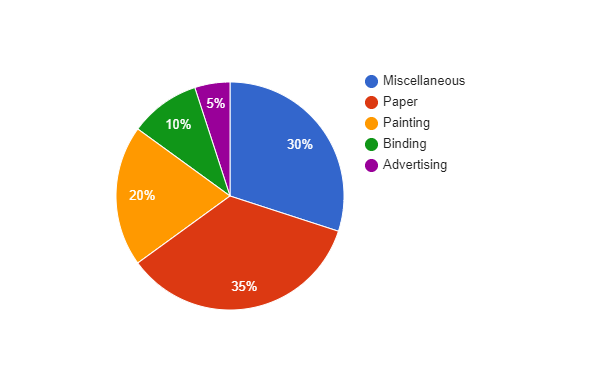### Question 7. Percentage of the different products of a village in a particular district are given below. Draw a pie chart representing this information.

Solution:

Given that, total % = 100%

Therefore,

Central angle = (component value/100) × 360°

Now the central angle for each activity will be calculated as follows :

Steps to construct pie chart using above data :

Step 1. Draw the circle of an appropriate radius.

Step 2. Now draw a vertical radius anywhere inside the circle.

Step 3. Now choose the largest central angle. Construct a sector of central angle, whose one radius coincides with the radius drawn in step 2 and the other radius is in clockwise direction to the vertical radius.

Step 4. Construct other sectors representing other values in clockwise direction in descending order of values of their central angles.

Step 5. Shade the sectors so obtained by different colors and label them.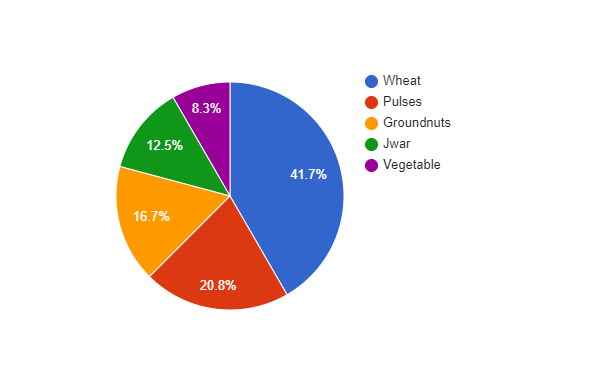### Question 8. Draw a pie diagram for the following data of expenditure pattern in a family :

Solution:

Given that, total % = 100%

Therefore,

Central angle = (component value/100) × 360°

Now the central angle for each activity will be calculated as follows :

Steps to construct pie chart using above data :

Step 1. Draw the circle of an appropriate radius.

Step 2. Now draw a vertical radius anywhere inside the circle.

Step 3. Now choose the largest central angle. Construct a sector of central angle, whose one radius coincides with the radius drawn in step 2 and the other radius is in clockwise direction to the vertical radius.

Step 4. Construct other sectors representing other values in clockwise direction in descending order of values of their central angles.

Step 5. Shade the sectors so obtained by different colors and label them.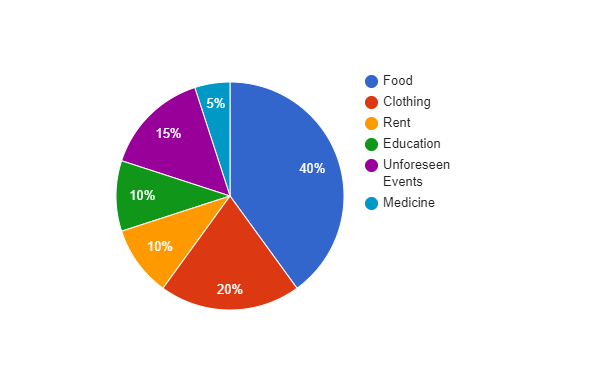### Question 9. Draw a pie diagram of the areas of continents of the world given in the following table:

Solution:

Given that, total Area = 133.3 million sq. km

Therefore,

Central angle = (component value/133.3) × 360°

Now the central angle for each activity will be calculated as follows :

Steps to construct pie chart using above data :

Step 1. Draw the circle of an appropriate radius.

Step 2. Now draw a vertical radius anywhere inside the circle.

Step 3. Now choose the largest central angle and construct a sector of central angle, whose one radius coincides with the radius drawn in step 2 and the other radius is in clockwise direction to the vertical radius.

Step 4. Construct other sectors representing other values in clockwise direction in descending order of values of their central angles.

Step 5. Shade the sectors so obtained by different colors and label them.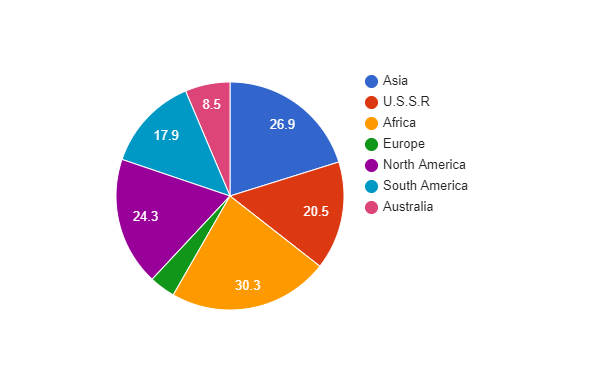### Question 10. The following data gives the amount spent on the construction of a house. Draw a pie diagram.

Solution:

Given that, total Expenditure = 300 thousand rupees

Therefore,

Central angle = (component value/300) × 360°

Now the central angle for each activity will be calculated as follows :

Steps to construct pie chart using above data :

Step 1. Draw the circle of an appropriate radius.

Step 2. Now draw a vertical radius anywhere inside the circle.

Step 3. Now choose the largest central angle and construct a sector of central angle, whose one radius coincides with the radius drawn in step 2 and the other radius is in clockwise direction to the vertical radius.

Step 4. Construct other sectors representing other values in clockwise direction in descending order of values of their central angles.

Step 5. Shade the sectors so obtained by different colors and label them.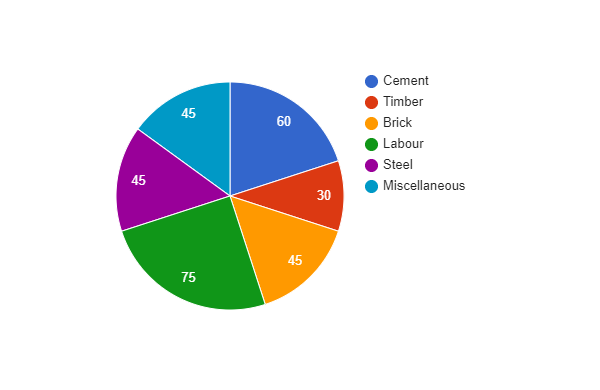My Personal Notes arrow_drop_up